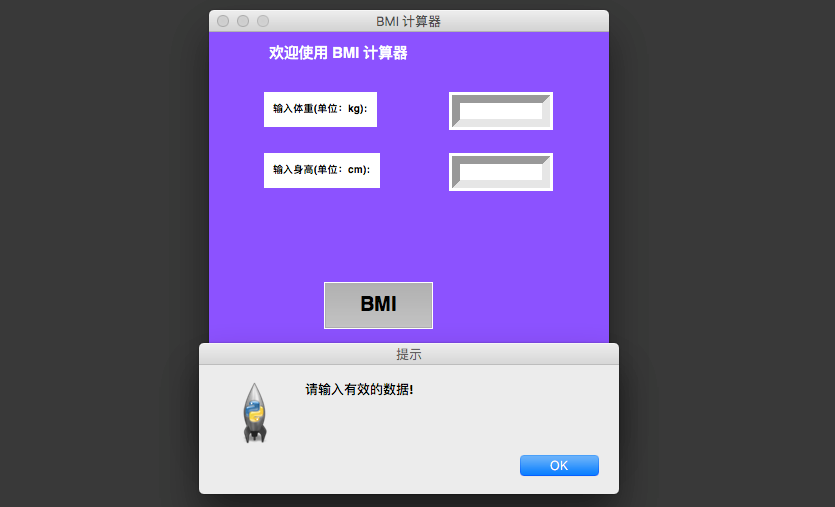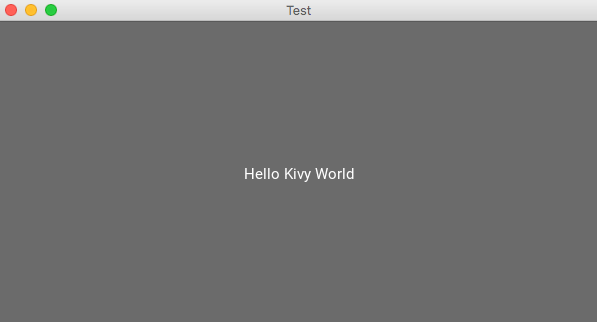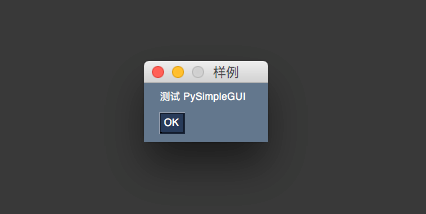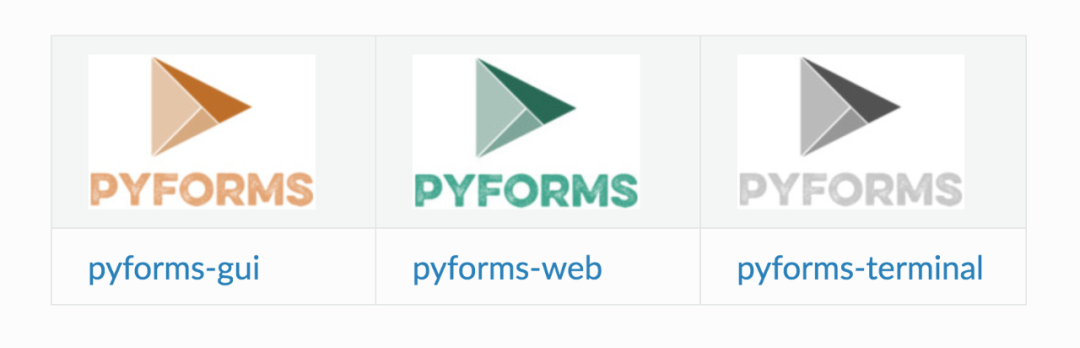# 七个Python必备的GUI库

GUI(图形用户界面)，顾名思义就是用图形的方式，来显示计算机操作的界面，更加方便且直观。

## 01. PyQt5

PyQt5由Riverbank Computing开发。基于Qt框架构建，是一个跨平台框架，可以给各种平台创建应用程序，包括：Unix、Windows、Mac OS。

PyQt将Qt和Python结合在一起。它不只是一个GUI工具包。还包括了线程，Unicode，正则表达式，SQL数据库，SVG，OpenGL，XML和功能完善的Web浏览器，以及许多丰富的GUI小部件集合。

 1 2 `# 安装PyQt5` `pip ``install` `-i https:``//pypi``.tuna.tsinghua.edu.cn``/simple` `PyQt5`

 1 2 3 4 5 6 7 8 9 10 11 12 13 14 15 16 17 18 19 20 21 22 `import` `sys` `from` `PyQt5.QtWidgets ``import` `QApplication, QWidget, QLabel, QVBoxLayout` `# 建立application对象` `app ``=` `QApplication(sys.argv)` `# 建立窗体对象` `w ``=` `QWidget()` `# 设置窗体大小` `w.resize(``500``, ``500``)` `# 设置样式` `w.layout ``=` `QVBoxLayout()` `w.label ``=` `QLabel(``"Hello World!"``)` `w.label.setStyleSheet(``"font-size:25px;margin-left:155px;"``)` `w.setWindowTitle(``"PyQt5 窗口"``)` `w.layout.addWidget(w.label)` `w.setLayout(w.layout)` `# 显示窗体` `w.show()` `# 运行程序` `sys.exit(app.exec_())`https://riverbankcomputing.com/software/pyqt/intro

https://www.guru99.com/pyqt-tutorial.html

## 02. Tkinter

Tkinter是Python中最受欢迎的GUI库之一。由于它简单易学的语法，成为GUI开发初学者的首选之一。

Tkinter提供了各种小部件，例如标签，按钮，文本字段，复选框和滚动按钮等。

 1 2 `# 安装tkinter` `pip ``install` `-i https:``//pypi``.tuna.tsinghua.edu.cn``/simple` `tkinter`

 1 2 3 4 5 6 7 8 9 10 11 12 13 14 15 16 17 18 19 20 21 22 23 24 25 26 27 28 29 30 31 32 33 34 35 36 37 38 39 40 41 42 43 44 45 46 47 48 49 50 51 52 53 54 55 `from` `tkinter ``import` `*` `from` `tkinter ``import` `messagebox` `def` `get_height():` `    ``# 获取身高数据(cm)` `    ``height ``=` `float``(ENTRY2.get())` `    ``return` `height` `def` `get_weight():` `    ``# 获取体重数据(kg)` `    ``weight ``=` `float``(ENTRY1.get())` `    ``return` `weight` `def` `calculate_bmi():` `    ``# 计算BMI系数` `    ``try``:` `        ``height ``=` `get_height()` `        ``weight ``=` `get_weight()` `        ``height ``=` `height ``/` `100.0` `        ``bmi ``=` `weight ``/` `(height ``*``*` `2``)` `    ``except` `ZeroDivisionError:` `        ``messagebox.showinfo(``"提示"``, ``"请输入有效的身高数据!!"``)` `    ``except` `ValueError:` `        ``messagebox.showinfo(``"提示"``, ``"请输入有效的数据!"``)` `    ``else``:` `        ``messagebox.showinfo(``"你的BMI系数是: "``, bmi)` `if` `__name__ ``=``=` `'__main__'``:` `    ``# 实例化object，建立窗口TOP` `    ``TOP ``=` `Tk()` `    ``TOP.bind(``""``, calculate_bmi)` `    ``# 设定窗口的大小(长 * 宽)` `    ``TOP.geometry(``"400x400"``)` `    ``# 窗口背景颜色` `    ``TOP.configure(background``=``"#8c52ff"``)` `    ``# 窗口标题` `    ``TOP.title(``"BMI 计算器"``)` `    ``TOP.resizable(width``=``False``, height``=``False``)` `    ``LABLE ``=` `Label(TOP, bg``=``"#8c52ff"``, fg``=``"#ffffff"``, text``=``"欢迎使用 BMI 计算器"``, font``=``(``"Helvetica"``, ``15``, ``"bold"``), pady``=``10``)` `    ``LABLE.place(x``=``55``, y``=``0``)` `    ``LABLE1 ``=` `Label(TOP, bg``=``"#ffffff"``, text``=``"输入体重(单位：kg):"``, bd``=``6``,` `                   ``font``=``(``"Helvetica"``, ``10``, ``"bold"``), pady``=``5``)` `    ``LABLE1.place(x``=``55``, y``=``60``)` `    ``ENTRY1 ``=` `Entry(TOP, bd``=``8``, width``=``10``, font``=``"Roboto 11"``)` `    ``ENTRY1.place(x``=``240``, y``=``60``)` `    ``LABLE2 ``=` `Label(TOP, bg``=``"#ffffff"``, text``=``"输入身高(单位：cm):"``, bd``=``6``,` `                   ``font``=``(``"Helvetica"``, ``10``, ``"bold"``), pady``=``5``)` `    ``LABLE2.place(x``=``55``, y``=``121``)` `    ``ENTRY2 ``=` `Entry(TOP, bd``=``8``, width``=``10``, font``=``"Roboto 11"``)` `    ``ENTRY2.place(x``=``240``, y``=``121``)` `    ``BUTTON ``=` `Button(bg``=``"#000000"``, fg``=``'#ffffff'``, bd``=``12``, text``=``"BMI"``, padx``=``33``, pady``=``10``, command``=``calculate_bmi,` `                    ``font``=``(``"Helvetica"``, ``20``, ``"bold"``))` `    ``BUTTON.grid(row``=``5``, column``=``0``, sticky``=``W)` `    ``BUTTON.place(x``=``115``, y``=``250``)` `    ``TOP.mainloop()`## 03. Kivy

Kivy是另一个开源的Python库，最大的优点就是可以快速地编写移动应用程序(手机)。

Kivy可以在不同的平台上运行，包括Windows、Mac OS、Linux、Android、iOS和树莓派。

 1 2 `# 安装kivy` `pip ``install` `-i https:``//pypi``.tuna.tsinghua.edu.cn``/simple` `kivy`

 1 2 3 4 5 6 7 8 `from` `kivy.app ``import` `App` `from` `kivy.uix.button ``import` `Button` `class` `TestApp(App):` `    ``def` `build(``self``):` `        ``return` `Button(text``=``" Hello Kivy World "``)` `TestApp().run()`## 04. wxPython

wxPython是一个跨平台GUI的Python库，可轻松创建功能强大稳定的GUI，毕竟是用C++编写的～

 1 2 `# 安装wxPython` `pip install ``-``i https:``/``/``pypi.tuna.tsinghua.edu.cn``/``simple wxPython`

 1 2 3 4 5 6 7 `import` `wx` `myapp ``=` `wx.App()` `init_frame ``=` `wx.Frame(parent``=``None``, title``=``'WxPython 窗口'``)` `init_frame.Show()` `myapp.MainLoop()`## 05. PySimpleGUI

PySimpleGUI也是基于Python的GUI框架。可以轻松制作自定义的GUI。

Remi将应用程序的界面转换为HTML，以便在Web浏览器中呈现。

 1 2 `# 安装PySimpleGUI` `pip install ``-``i https:``/``/``pypi.tuna.tsinghua.edu.cn``/``simple PySimpleGUI`

 1 2 3 4 5 6 7 8 9 `import` `PySimpleGUI as sg` `layout ``=` `[[sg.Text(``"测试 PySimpleGUI"``)], [sg.Button(``"OK"``)]]` `window ``=` `sg.Window(``"样例"``, layout)` `while` `True``:` `    ``event, values ``=` `window.read()` `    ``if` `event ``=``=` `"OK"` `or` `event ``=``=` `sg.WIN_CLOSED:` `        ``break` `window.close()`## 06. PyGUI

PyGUI是一个以简单API而闻名的GUI框架，减少Python应用与平台底层GUI之间的代码量。

Python2和Python3，都是可以支持的。

https://www.cosc.canterbury.ac.nz/greg.ewing/python_gui/

https://realpython.com/pysimplegui-python/

## 07. Pyforms

Pyforms是用于开发GUI应用程序的一个跨平台框架。pyforms是一个Python2.7/3.x跨环境图形应用开发框架，模块化和代码复用可以节省大量工作。

 1 2 `# 安装PyFroms` `pip install ``-``i https:``/``/``pypi.tuna.tsinghua.edu.cn``/``simple PyFroms`